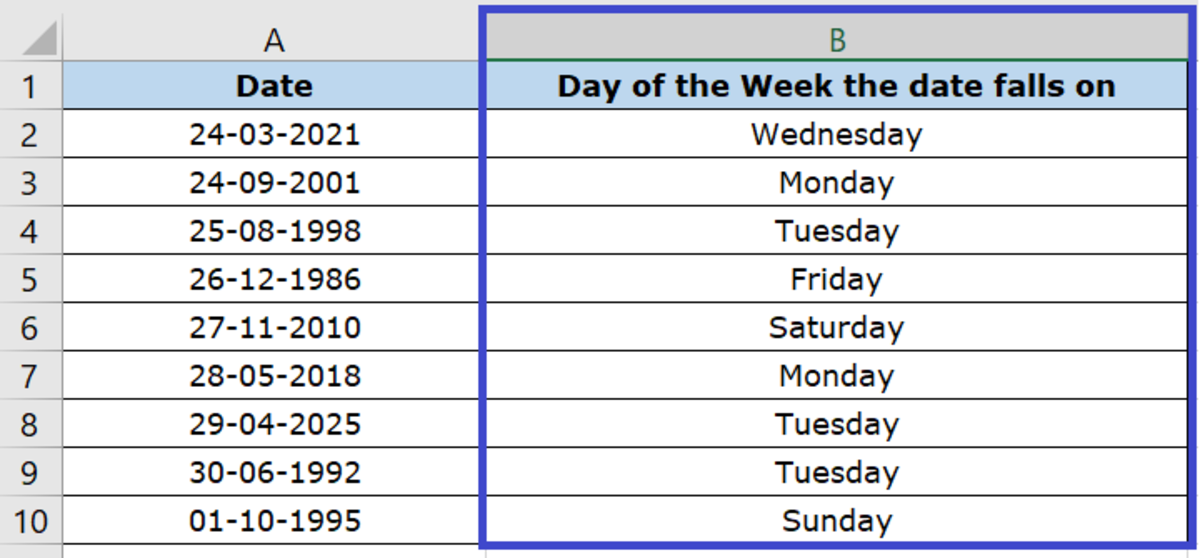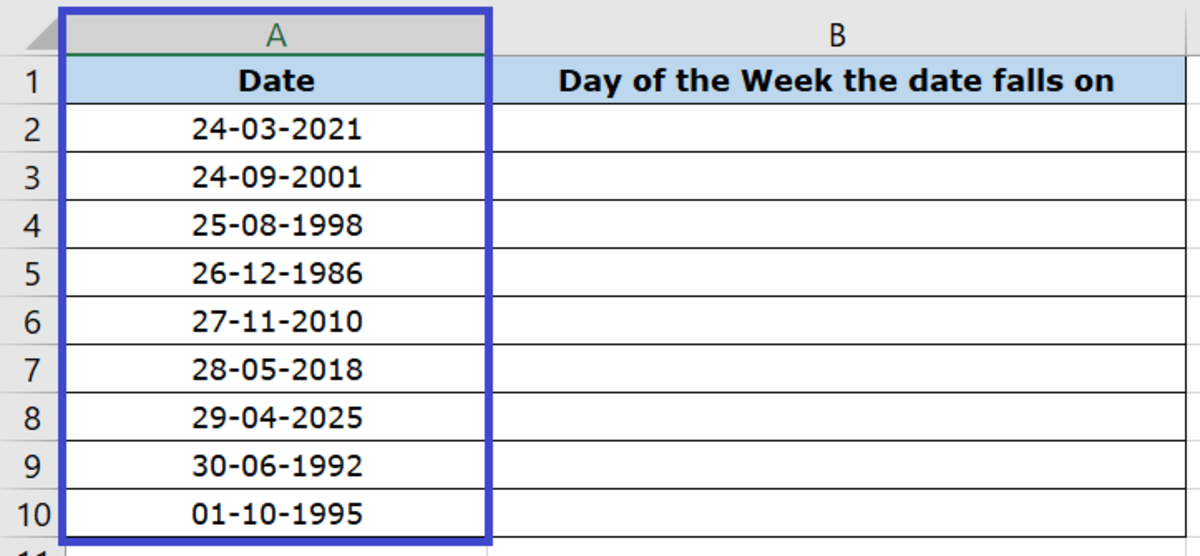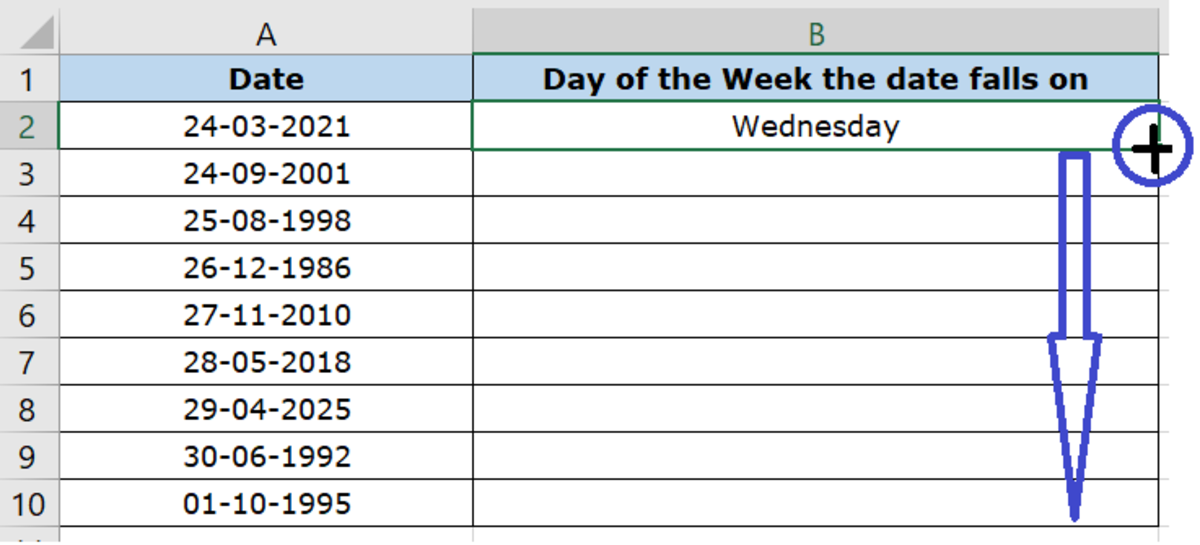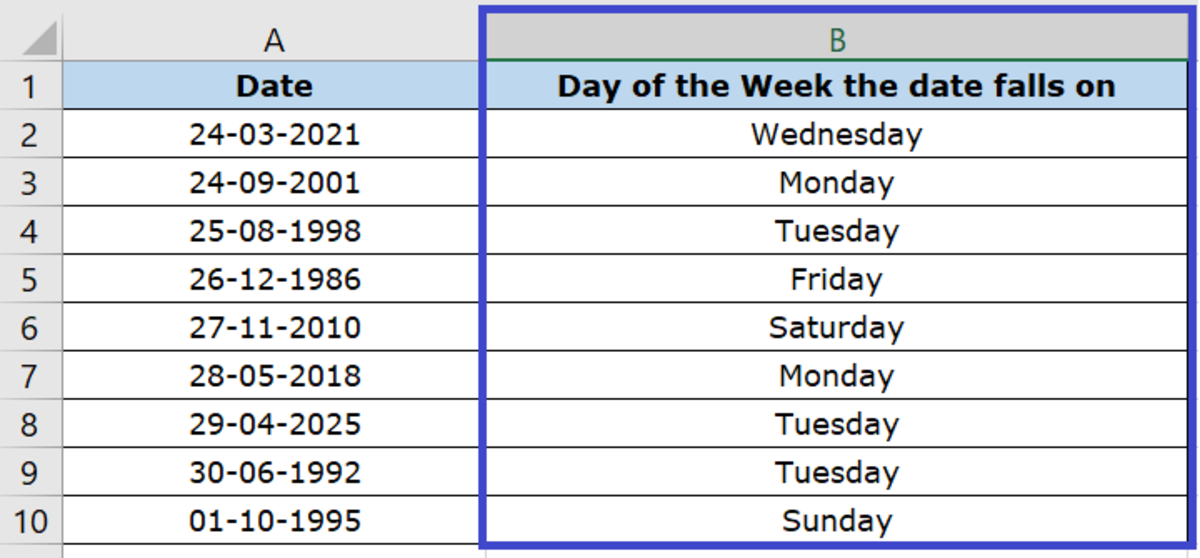# How to Convert Dates to Days of the Week in Excel

Neha is a software professional with 13+ years of experience in the IT Industry. She enjoys writing technical tutorials.How to Dynamically Convert a Given Date to the Day of the Week it Falls on

## How Do I Dynamically Convert Dates to the Days of the Week? (3 Easy Steps)

Are you looking to easily and dynamically convert a set of given dates to the days of the week they fall on using Microsoft Excel?

Here's my step-by-step tutorial with easy steps for you to follow.

### 1. Identify the Column to be Used to Convert the Dates to the Days of the Week

Let us assume we have a list of dates in a Microsoft Excel Worksheet and we would like to dynamically convert the dates to the days of the week they fall on.

Here's the sample data we are going to use to demonstrate how to do this.

In our table here, we have a list of dates in the (DD-MM-YYYY) format and we are going to use Microsoft Excel Formulas to dynamically convert them to days of the week.

So in this case, the column to be used to determine these values would be Column A, which is holding the date values.Column A holds the dates to be converted

### 2. Apply the Formula in the Cell Where You Want the Converted Value to Appear

Formula to be entered in cell: B2 (That's where the Day of the Week should appear).

Formula to be used: +TEXT (value, format_text)—Converts a value to text in a specific number format.

Scroll to Continue

Keep in mind:

• Value is the cell that holds the date value. In this case it is A2.
• Format_text is the format in which the day should appear. In our case we have used "DDDD" as the format_text, which results in it appearing as the full name of the day of the week. For example, Wednesday! Our formula becomes: +TEXT(A2, "DDDD")
• If we use "DDD" as the format_text instead, the result will be the day of the week in shortened form (the first three letters). For example, Wed!
• If we use "DD" as the format_text instead, the result will be the day of the week in number form. For example, 24 (meaning the 24th day of the month).

### 3. Copy the Formula to the Remaining Cells

You could either copy and paste the formula in the remaining cells, or you could drag the plus sign at the bottom right corner of the cell so that the formula gets copied to the remaining cells.Copy the formula to the remaining cells

### And Voilà!

Once the formula is copied to the other cells, it automatically converts the values from the entire list of the dates.

The result is the days of the week in each of the dates in the selected column falls on.Dates dynamically converted to Days of the Week

This content is accurate and true to the best of the author’s knowledge and is not meant to substitute for formal and individualized advice from a qualified professional.

© 2021 Petite Hubpages Fanatic